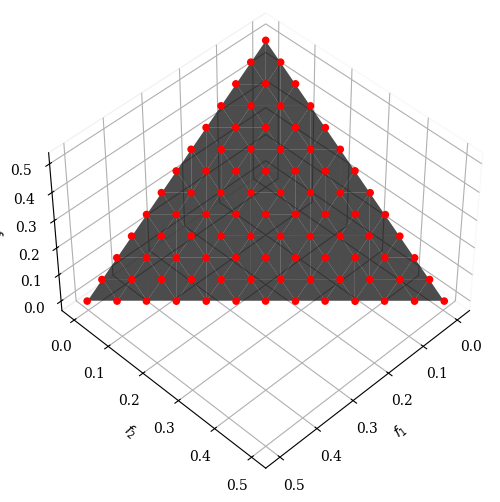Version: 0.6.0

# RVEA: Reference Vector Guided Evolutionary Algorithm¶

The algorithm is implemented based on . In RVEA, a scalarization approach, termed angle penalized distance (APD), is adopted to balance the convergence and diversity of the solutions in the high-dimensional objective space. Furthermore, an adaptation strategy is proposed to dynamically adjust the reference vectors’ distribution according to the objective functions’ scales. An illustration of the APD is shown below:## Example¶

Info

Note that the APD is adapted based on the progress the algorithm has made. Thus, termination criteria such as n_gen or n_evals should be used.

:

from pymoo.algorithms.moo.rvea import RVEA
from pymoo.optimize import minimize
from pymoo.problems import get_problem
from pymoo.util.ref_dirs import get_reference_directions
from pymoo.visualization.scatter import Scatter

problem = get_problem("dtlz1", n_obj=3)

ref_dirs = get_reference_directions("das-dennis", 3, n_partitions=12)

algorithm = RVEA(ref_dirs)

res = minimize(problem,
algorithm,
termination=('n_gen', 400),
seed=1,
verbose=False)

plot = Scatter()
plot.show()

:

<pymoo.visualization.scatter.Scatter at 0x7feb1bfac430>## API¶

class pymoo.algorithms.moo.rvea.RVEA(self, ref_dirs, alpha=2.0, adapt_freq=0.1, pop_size=None, sampling=FloatRandomSampling(), selection=RandomSelection(), crossover=SBX(eta=30, prob=1.0), mutation=PM(eta=20), eliminate_duplicates=True, n_offsprings=None, output=MultiObjectiveOutput(), **kwargs)
Parameters
ref_dirsnumpy.array

The reference direction that should be used during the optimization. Each row represents a reference line and each column a variable.

Defines the ratio of generation when the reference directions are updated.

pop_sizeint (default = None)

By default the population size is set to None which means that it will be equal to the number of reference line. However, if desired this can be overwritten by providing a positive number.

sampling

The sampling process defines the initial set of solutions which are the starting point of the optimization algorithm. Here, you have three different options by passing

(i) A Sampling implementation which is an implementation of a random sampling method.

(ii) A Population object containing the variables to be evaluated initially OR already evaluated solutions (F needs to be set in this case).

(iii) Pass a two dimensional numpy.array with (n_individuals, n_var) which contains the variable space values for each individual.

selectionSelection

This object defines the mating selection to be used. In an evolutionary algorithm each generation parents need to be selected to produce new offsprings using different recombination and mutation operators. Different strategies for selecting parents are possible e.g. selecting them just randomly, only in the neighborhood, using a tournament selection to introduce some selection pressure, …

crossoverCrossover

The crossover has the purpose of create offsprings during the evolution. After the mating selection the parents are passed to the crossover operator which will dependent on the implementation create a different number of offsprings.

mutationMutation

Some genetic algorithms rely only on the mutation operation. However, it has shown that increases the performance to perform a mutation after creating the offsprings through crossover as well. Usually the mutation operator needs to be initialized with a probability to be executed. Having a high probability of mutation will most of the time increase the diversity in the population.

eliminate_duplicatesbool

The genetic algorithm implementation has a built in feature that eliminates duplicates after merging the parent and the offspring population. If there are duplicates with respect to the current population or in the offsprings itself they are removed and the mating process is repeated to fill up the offsprings until the desired number of unique offsprings is met.

n_offspringsint (default: None)

Number of offspring that are created through mating. By default n_offsprings=None which sets the number of offsprings equal to the population size. By setting n_offsprings=1 a, so called, steady-state version of an algorithm can be achieved.